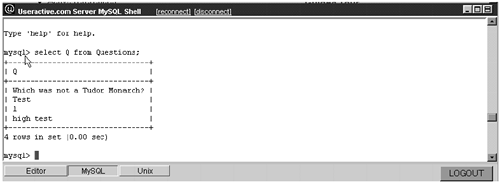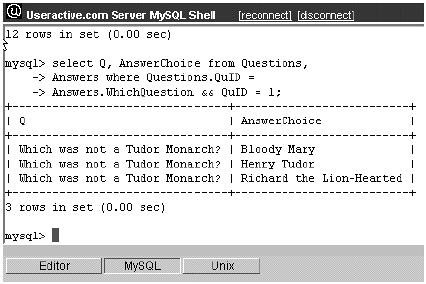# Reading Records from the Table

Of course, storing data is useless unless we can recover it. MySQL gives us a powerful assortment of tools to retrieve and organize data.

#### Select

When we want to query the database and retrieve data from the tables (or collect data from anywhere else, for that matter), we do so with a SELECT statement. The simplest syntax is

##### MySQL
` mysql> SELECT  <columns_of_output>; `

We can use this very simple form to get MySQL system information. So, for instance,

##### MySQL
` mysql> SELECT now(), version(); `

yields a two-column result where the current time and date as well as version information are displayed.

##### Output
` +---------------------+-----------+  now()                version()  +---------------------+-----------+  2000-09-17 08:00:15  3.22.23b   +---------------------+-----------+ `

#### From

SELECT is most useful, of course, when querying the database. This requires us to identify the tables from which we are selecting data. To retrieve data from a table, we say

##### MySQL
` SELECT  <* or columns of output> FROM  <table>; `

Example:

##### MySQL
` mysql> SELECT Q FROM Questions; `

returns the text (column Q ) of each entry in the Question database (Figure 12.2).

##### Figure 12.2. Using the Editor to Select the Q Column from Our Questions Table#### Where

We generally want to apply constraints to the data returned from SELECT . The most straightforward contraint is the WHERE clause, which tests each row against a conditional to see whether or not it will be filtered out. Conditionals use familiar boolean comparisons (listed in Table 12.9) and logical connectors to test column contents against constants, other columns, or SQL functions.

##### Table 12.9. Conditional Operators
 = equals <=> NULL safe equals <> != not equals <[=] Less than [or equal] >[=] Greater than [or equal] IS [NOT] NULL ISNULL(expr) test for NULL BETWEEN min AND max within bounds? expr [NOT] IN (value,...) member of set? INTERVAL(N,N1,N2,N3,...) in ascending order? [NOT] LIKE SQL wildcard pattern match: % matches anything or nothing _ matches any single character [NOT] REGEXP [NOT] RLIKE full regular expression test STRCMP(expr1,expr2) Zero when strings match
##### MySQL
` SELECT  <data> FROM  <table> WHERE  <conditional>; `

Example:

##### MySQL
` mysql> SELECT Q FROM Questions WHERE Author=1 ; `

returns the text of all questions written by Player 1.

#### Group By

Often it is useful to collect records in groups that share a common property. This feature combines all records with equal values in the same column.

##### MySQL
` SELECT  <data> FROM  <table> GROUP BY  <column(s)>; `

Example:

##### MySQL
` mysql> SELECT Author, count(*) FROM Questions GROUP BY Author ; `

returns each author and a count of the group of questions that he has written.

#### Having

The WHERE command balks at conditionals that have functions in them. WHERE would be unable to meet our needs if we decide to restrict the records returned based on the count() function in the previous example, so that only authors with more than 3 questions were displayed. HAVING fills this void. HAVING can use columns that are constructed using GROUP BY and that use functions such as count() .

##### MySQL
` mysql> SELECT Author, count(*) FROM Questions GROUP BY Author     -> HAVING count(*)>= 2; `

returns a table with all the authors who have submitted two or more questions.

Although in theory we could always use HAVING to replace WHERE , standard practice restricts the use of HAVING to those queries where its unique capabilities are required.

#### Order By

MySQL returns a table of data in the useless order of chronological creation. We can change this using the ORDER BY command, which takes as a parameter any number of columns. The table is then organized in alphabetic or numeric order (depending on the data stored in the column). After sorting based on the first column, ORDER BY uses the next parameter to break any ties, and so on until, after all parameters have been used, the creation order breaks any remaining ties.

##### MySQL
` mysql> SELECT * FROM Answers ORDER BY WhichQuestion; `

returns the entire Answers table, with all the answers to each question grouped together and the groups organized from lowest WhichQuestion to highest.

In case we wish to reverse the order, we can do so by adding the DESC keyword after the column name . The DESC keyword affects only the column it immediately follows .

##### MySQL
` mysql> SELECT * FROM Answers ORDER BY WhichQuestion DESC, AnswerChoice; `

returns all the answers sorted first in reverse order (highest to lowest) of WhichQuestion and second based on alphabetic order of AnswerChoice s.

The sequence of these constraints in the SELECT query line is critical. They must be applied like this:

##### MySQL
` mysql> SELECT  <data>       ->        FROM <table>       ->        WHERE <conditional>       ->        GROUP BY <column<s)>       ->        HAVING <conditional>       ->        ORDER BY <column(s)>       ->        ; `

#### Alias

It is often useful to rename data as it is assembled by a query. The alias AS command allows us to create virtual columns and tables. This makes self-joins easy and also lets us smooth the transition from SQL database to XML datagram.

##### MySQL
` SELECT  <data>  AS <alias> SELECT  <data>  FROM <table> AS <alias> `

Example:

##### MySQL
` mysql>  SELECT Author, COUNT(*) AS x     ->   FROM Questions GROUP BY Author ORDER BY x; `

This code collects each author's questions into a group, counts them, and then uses the count (called x ) to sort the result and present a list of each author's questions in order of how prolific each author is. Note WHERE , unlike GROUP BY , HAVING and ORDER BY cannot refer to a column by its alias.

#### Joins

Joins ”as explained in depth in the previous chapter ”are useful tools for combining data from more than a single table into one virtual table. In a well-used relational database, the contents of an object are distributed over several tables, and it is usually necessary to fold the data together with a join. This necessity is particularly true with XML objects, whose tree-like structure does not map easily to the rows and columns of an individual table.

MySQL allows us two types of joins. We are allowed to use both the inner join and the outer join. However, our outer joins are limited to the use of the left outer join. This restriction should be no problem, since we learned last chapter that a right outer join can be turned into a left outer join by switching the order of the tables.

We will need to refer to various columns in order to refer to specific records and match keys. We can do this one of two ways. If the column name is unique across the tables, we can simply use the column name. Otherwise, we simply refer to it by the name of the table and the name of the column separated by a period (<TableName>.<ColumnName>). We cannot use the alias of a column. However, if we are performing a self-join and use an alias to represent a table, we must use only the alias to reference the table.

If we wish to perform an inner join, we are allowed to be creative. There are several commands we can use to accomplish the join. CROSS JOIN , STRAIGHT JOIN , JOIN or simply ',' all indicate an inner join. We use the WHERE command to specify the conditions for syncing up keys. The various formats are

##### MySQL
` mysql> SELECT Q, AnswerChoice FROM Questions JOIN Answers WHERE QuID =     -> WhichQuestion; mysql> SELECT Q, AnswerChoice FROM Questions CROSS JOIN Answers WHERE        QuID = -> WhichQuestion; mysql> SELECT Q, AnswerChoice FROM Questions STRAIGHT JOIN Answers WHERE        QuID = -> WhichQuestion; mysql> SELECT Q, AnswerChoice FROM Questions, Answers WHERE QuID =     -> WhichQuestion; `

In order to add the power to specify rows, we can add more conditions to the WHERE clause using an AND . So, if we wish to join the Questions and Answers tables, but are only concerned about a question with a QuID of 1, the command and output would look just like they do in Figure 12.3.

##### Figure 12.3. Screenshot of a JOIN in MySQLIf, on the other hand, we wish to perform an outer join (a left outer join, since that is all we are allowed), we are not given so many choices of syntax. The command simply is

##### MySQL
` mysql> SELECT * FROM <TableA> LEFT JOIN <TableB> ON <TableA>.<Column> =      -> <TableB>.<Column>; `

A WHERE statement can follow the ON command to further specify rows.

##### MySQL
` mysql> SELECT * FROM <TableA> LEFT JOIN <TableB> ON <TableA>.<Column> =      -> <TableB>.<Column> WHERE <condition>; `Flash and XML[c] A Developer[ap]s Guide
ISBN: 201729202
EAN: N/A
Year: 2005
Pages: 160